# Common Core: 6th Grade Math : Compare the Measure of Center and Measure of Variation: CCSS.Math.Content.6.SP.A.3

## Example Questions

← Previous 1

### Example Question #1 : Compare The Measure Of Center And Measure Of Variation: Ccss.Math.Content.6.Sp.A.3

Select the description that best describes the measure of center for a numerical data set.

The measure of center is the part of a graph or data set that contains the highest valued point

The measure of center is the part of a graph or data set that contains the most points

The measure of center is a value at the center of a data set and summarizes all of the values in a data set with a single number

The measure of center is a value at the center of a data set that describes how all of the values in a data set vary with a single number

The measure of center is a value at the center of a data set and summarizes all of the values in a data set with a single number

Explanation:

By definition, the measure of center for a numerical data set is a value at the center of a data set and summarizes all of the values in a data set with a single number. Algebraically, the most common ways to solve for the measure of center is to solve for the mean or median.

### Example Question #1 : Compare The Measure Of Center And Measure Of Variation: Ccss.Math.Content.6.Sp.A.3

Select the description that best describes the measure of variation for a numerical data set.

The measure of variation is the part of a graph or data set that contains the most points

The measure of variation describes how the data set's values vary with a single number.

The measure of variation is the part of a graph or data set that contains the highest valued point

The measure of variation is a value at the center of a data set and summarizes all of the values in a data set with a single number

The measure of variation describes how the data set's values vary with a single number.

Explanation:

By definition, the measure of variation describes how the data set's values vary with a single number.

### Example Question #1 : Compare The Measure Of Center And Measure Of Variation: Ccss.Math.Content.6.Sp.A.3

Mr. Sommers wanted to see how much money his students spent on school lunch in a given day. The distribution of this data is shown in the plot provided. Use mean to solve for the center of this distribution.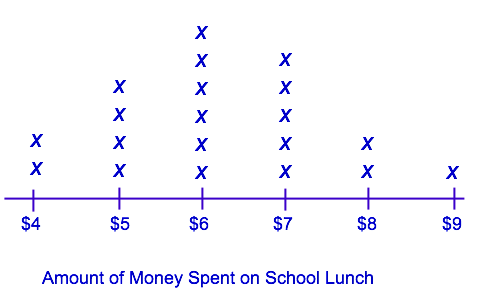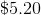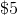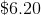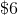Explanation:

In order to solve for the mean, we need to add up all of the money spent on lunch, and then divide by the number of addends in our set: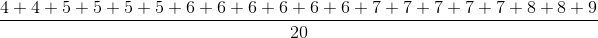The center of distribution, using the mean, is### Example Question #1 : Compare The Measure Of Center And Measure Of Variation: Ccss.Math.Content.6.Sp.A.3

Mr. Sommers wanted to see how much money his students spent on school lunch in a given day. The distribution of this data is shown in the plot provided. Use median to solve for the center of this distribution.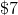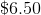Explanation:

In order to solve for median, we need to list all of our data points in order from least to greatest: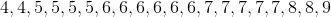Next, we want to find the number, or numbers that are in the middle: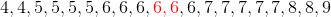The center of distribution, using the median, is### Example Question #1 : Compare The Measure Of Center And Measure Of Variation: Ccss.Math.Content.6.Sp.A.3

Mr. Sommers wanted to see how much money his students spent on school lunch in a given day. The distribution of this data is shown in the plot provided. What is the highest amount of money spent on lunch by one of Mr. Sommers's students?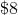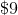Explanation:

The plot shows the amount of money spent on school lunch on a number line.is the greatest number on the number line, and one student spenton lunch; thus,is the correct answer.

### Example Question #1 : Compare The Measure Of Center And Measure Of Variation: Ccss.Math.Content.6.Sp.A.3

Mr. Sommers wanted to see how much money his students spent on school lunch in a given day. The distribution of this data is shown in the plot provided. What is the least amount of money spent on lunch by one of Mr. Sommers's students?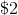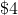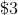Explanation:

The plot shows the amount of money spent on school lunch on a number line.is the smallest number on the number line, and two students spenton lunch; thus,is the correct answer.

### Example Question #1 : Compare The Measure Of Center And Measure Of Variation: Ccss.Math.Content.6.Sp.A.3

Mr. Sommers wanted to see how much money his students spent on school lunch in a given day. The distribution of this data is shown in the plot provided. Use the information provided in the plot to fill in the blank of the table.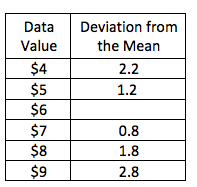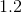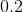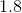Explanation:

The table shows how far away each data value is from the mean. In order to solve for the missing piece of the table, we first need to solve for the mean:

In order to solve for the mean, we need to add up all of the money spent on lunch, and then divide by the number of addends in our set: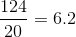The mean for this data set isNext, we can subtract the data value from the mean to find the deviation from the mean: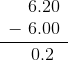The correct answer is### Example Question #31 : Statistics & Probability

Mr. Sommers wanted to see how much money his students spent on school lunch in a given day. The distribution of this data is shown in the plot provided. Use the information provided in the plot to fill in the blank of the table.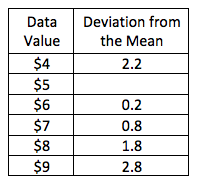Explanation:

The table shows how far away each data value is from the mean. In order to solve for the missing piece of the table, we first need to solve for the mean:

In order to solve for the mean, we need to add up all of the money spent on lunch, and then divide by the number of addends in our set:The mean for this data set isNext, we can subtract the data value from the mean to find the deviation from the mean: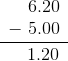The correct answer is### Example Question #9 : Compare The Measure Of Center And Measure Of Variation: Ccss.Math.Content.6.Sp.A.3

Mr. Sommers wanted to see how much money his students spent on school lunch in a given day. The distribution of this data is shown in the plot provided. Use the information provided in the plot to fill in the blank of the table.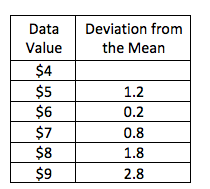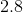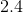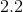Explanation:

The table shows how far away each data value is from the mean. In order to solve for the missing piece of the table, we first need to solve for the mean:

In order to solve for the mean, we need to add up all of the money spent on lunch, and then divide by the number of addends in our set:The mean for this data set isNext, we can subtract the data value from the mean to find the deviation from the mean: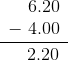The correct answer is### Example Question #2 : Compare The Measure Of Center And Measure Of Variation: Ccss.Math.Content.6.Sp.A.3

Mr. Sommers wanted to see how much money his students spent on school lunch in a given day. The distribution of this data is shown in the plot provided. Use the information provided in the plot to fill in the blank of the table.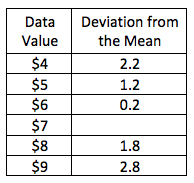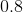Explanation:

The table shows how far away each data value is from the mean. In order to solve for the missing piece of the table, we first need to solve for the mean:

In order to solve for the mean, we need to add up all of the money spent on lunch, and then divide by the number of addends in our set:The mean for this data set isNext, we can subtract the data value from the mean to find the deviation from the mean: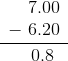The correct answer is← Previous 1

### All Common Core: 6th Grade Math Resources